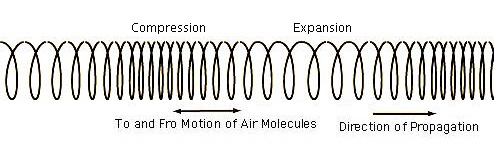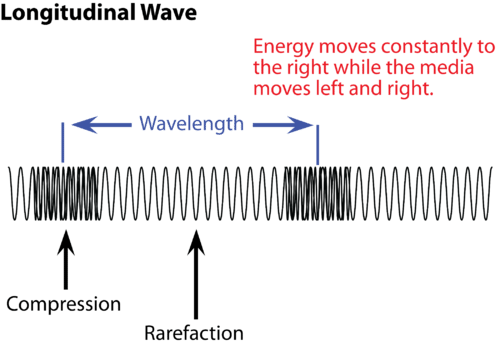wave types
label/draw waves
Wave equations
100what type of wave is this?

longitude wave

100

draw a picture of a transverse wave and label the crest.100

A wave has a wavelength of 20 meters and a wave speed of 260/s, what is the frequency of the wave?

show your work here:

260 = 20 * x

/20    /20

x= 13 Hz

200

A longitude wave consists of what? chose one.

A. crest and trough in the medium

B. compression and refraction in the medium

C. crest and trough in a vacuum

D. both A and B

B. compression and refraction in the medium

200

draw a picture of a transverse wave and label the trough.200

A wave has a frequency of 60 and a wavelength of 20 meters. What is the wave speed? show your work.

S= 60*20 =1200

300What type of wave is this?

Transverse wave

300

draw a longitudinal wave and label the rarefaction.300

A wave has a wavelength of 10 meters and a wave speed of 340 m/s what is the frequency of the wave? show your work.

340 = x*10

/10        /10

x = 34 hz

400

What happens when two tranverse waves meet?

They create a bigger wave.

400

draw and label the amplitude on a transverse wave400

What is the equation to find the frequency of a wave.

m/s = x* m

/m         /m

x = hz

500

What is the difference between a transverse wave and a longitudinal wave?

Transverse is a up and down motion while longitudinal wave is created by a push movement.

500

draw and label the compression and a wavelength of a longitudinal wave500

what is the velocity measured in?

Meters per second (ms2)

Click to zoom